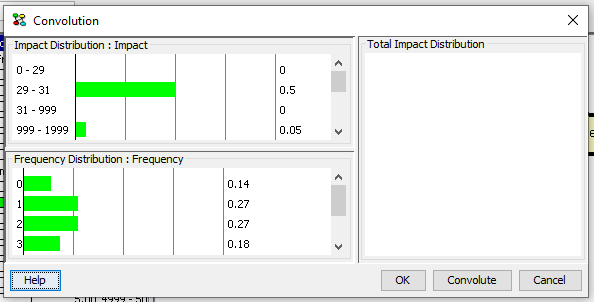# Convolution of Frequency-Impact Distributions¶

The convolution dialog allows the user to compute the convolution of frequency-impact distributions where the convolution is computed as a weighted sum of independent variables distributed as impact with the number of terms in the sum defined by frequency. The frequency node represents the probability distribution over the number of events.

## What is Convolution?¶

The assumption of the convolution functionality is that the frequency node predicts the expected number of events in one time period while the impact node predicts the cost of one given event. The frequency and impact nodes may dependent of the value of other nodes. The convolution of the frequency and impact distributions is the total impact. The convolution of a frequency distribution and an impact distribution is computed as the sum of independent variables as illustrated in this formula:

$P(F = 1) * I + P(F = 2) * (I + I) + P(F = 3) * (I + I + I) + ...$

where F is the frequency node and the I’s are independent random variables distributed as the impact node. The convolution is a sum of distributions where the kth term is the probability of k events multiplied with the distribution of a sum of k cost variables where each cost variable is a random variable with a distribution equal to that of impact and all cost variables are independent.

## Computing a Convolution¶

Convolution of frequency-impact distributions is supported for a pair nodes consisting of a numbered node and an interval node. The numbered and interval nodes must be well-formed. The interval node is well-formed if its intervals cover the range from zero to infinity (and it otherwise meets the requirements for an interval node). The numbered node is well-formed if its state values are zero, one, two, three, e.t.c. (and it otherwise meets the requirements for a numbered node). The numbered node is interpreted as the frequency node and the interval node is interpreted as the impact node. The convolution of the frequency and impact distributions is computed with respect to the states of an interval node. This is the total impact node. The intervals of the total impact node are equal to the intervals of the impact node.

The convolution algorithm is based on the Table Generator implementation, i.e., for each interval a predefined number of values are used in the calculations (as opposed to only using the middle value of an interval).

Convolution is supported for a domain in sum-normal equilibrium.

Figure 1 shows the convolution pane appearing after activating the convolution analysis. In this pane the result of convoluting the frequency and impact distributions is shown (Figure 2). Initially, Figure 1 shows the (posterior) probability distributions of the frequency node and the impact node.Figure 1: The dialog shows the distributions of the frequency node and the impact node.¶

The total impact distribution (i.e., the convolution of frequency and impact) is computed once the Convolute button is depressed.